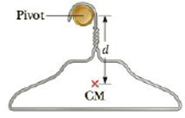Chapter 13, Problem 38P

Chapter
Section
Textbook Problem

A coat hanger of mass m = 0.238 kg oscillates on a peg as a physical pendulum as shown in Figure P13.38. The distance from the pivot to the center of mass of the coat hanger is d = 18.0 cm and the period of the motion is T = 1.25 s. Find the moment of inertia of the coat hanger about the pivot.To determine
The moment of inertia of the coat hanger about the pivot.

Explanation

Given info: The mass of the coat hanger is m=0.238kg . The distance of the pivot to the center of mass is d=18.0cm . The period of the motion is T=1.25s .

The time period of a physical pendulum is defined by,

T=2πImgL

• I is the moment of inertia of the object
• m is the mass of the object
• L is the distance between the pivot and center of mass of the object
• g is the free fall acceleration

Re-arranging for moment of inertia I ,

I=mgL(T2π)2

Substituting m=0.238kg , g=9.80ms-2 , T=1.25s and L=18.0cm

I=(0

Still sussing out bartleby?

Check out a sample textbook solution.

See a sample solution

The Solution to Your Study Problems

Bartleby provides explanations to thousands of textbook problems written by our experts, many with advanced degrees!

Get Started